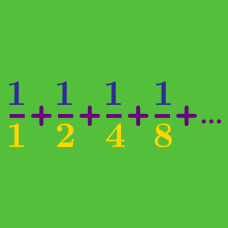Calculus

# Geometric Progression Sum

Evaluate

$1 + 2 + 2^2 + 2^3 + \ldots + 2^{ 24 }.$

A bookshelf has $6$ shelves. The top shelf has $2$ books, and each other shelf has $3$ times as many books as the shelf directly above it. If you want to read a book from the shelves, how many choices do you have?

Details and assumptions

All of the books are different.

The series $a_1, a_2, a_3, a_4, a_5, a_6, a_7$ is a geometric progression with $a_4 = 48$ and $a_5 = 96$. What is the sum of the these $7$ terms?

Details and assumptions

A geometric progression is a sequence of numbers, in which each subsequent number is obtained by multiplying the previous number by a common ratio / multiple. For example, $1, 2, 4, 8, 16, 32, 64, \ldots$ is a geometric progression with initial term 1 and common ratio 2.

Consider a geometric progression with initial term $4$ and common ratio $5$. What is the smallest value of $n$ for which the sum of the first $n$ terms is greater than $800$?

Evaluate the sum to infinity:

$\frac{ 14 } { 2^0} + \frac{ 14 } { 2^1 } + \frac{ 14 } { 2^2 } + \ldots .$

×